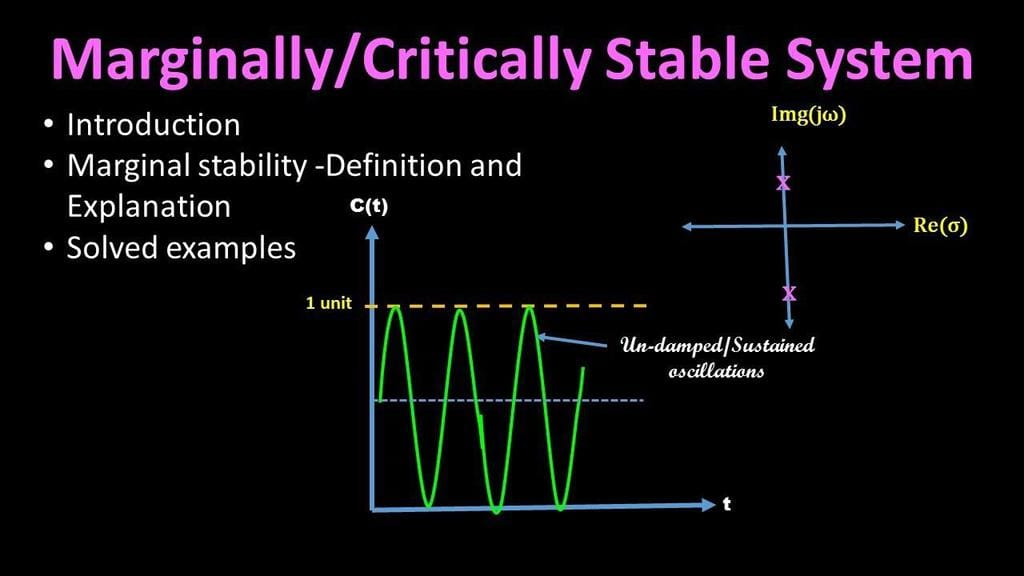# Test: Properties of LTI Systems

## 20 Questions MCQ Test Signals and Systems | Test: Properties of LTI Systems

Description
Attempt Test: Properties of LTI Systems | 20 questions in 15 minutes | Mock test for Electrical Engineering (EE) preparation | Free important questions MCQ to study Signals and Systems for Electrical Engineering (EE) Exam | Download free PDF with solutions
QUESTION: 1

### What is the rule h*(x+y) = (y+x)*h called?

Solution:

By definition, the commutative rule h*x=x*h.

QUESTION: 2

### Does the system h(t) = exp([-1-2j]t) correspond to a stable system?

Solution:

The system corresponds to an oscillatory system, this resolving to a marginally stable system.

QUESTION: 3

### What is the rule h*(x*c) = (x*h)*c called?

Solution:

By definition, the commutative rule i h*x=x*h and associativity rule = h*(x*c) = (h*x)*c.

QUESTION: 4

Is y[n] = n*cos(n*pi/4)u[n] a stable system?

Solution:

The ‘n’ term in the y[n] will dominate as it reaches to infinity, and hence could reach infinite values.

QUESTION: 5

What is the rule (h*x)*c = h*(x*c) called?

Solution:

By definition, the associativity rule = h*(x*c) = (h*x)*c.

QUESTION: 6

Is y[n] = n*sin(n*pi/4)u[-n] a stable system?

Solution:

The ‘n’ term in the y[n] will dominate as it reaches to negative infinity, and hence could reach infinite values. Eventhough + infinity would not be a problem, still the resultant system would be unstable.

QUESTION: 7

What is the following expression equal to: h*(c*(b+d(t))), d(t) is the delta function

Solution:

Apply commutative and associative rules

QUESTION: 8

Does the system h(t) = exp([1-4j]t) correspond to a stable system?

Solution:

The system corresponds to an unstable system, as the Re(exp) term is a positive quantity.

QUESTION: 9

The system transfer function and the input if exchanged will still give the same response.

Solution:

By definition, the commutative rule i h*x=x*h=y. Thus, the response will be the same.

QUESTION: 10

For an LTI discrete system to be stable, the square sum of the impulse response should be

Solution:

If the square sum is infinite, the system is an unstable system. If it is zero, it means h(t) = 0 for all t. However, this cannot be possible. Thus, it has to be finite.

QUESTION: 11

What is the rule h*x = x*h called?

Solution:

By definition, the commutative rule h*x=x*h.

QUESTION: 12

For an LTI discrete system to be stable, the square sum of the impulse response should be

Solution:

If the square sum is infinite, the system is an unstable system. If it is zero, it means h(t) = 0 for all t. However, this cannot be possible. Thus, it has to be finite.

QUESTION: 13

What is the rule (h*x)*c = h*(x*c) called?

Solution:

By definition, the associativity rule = h*(x*c) = (h*x)*c.

QUESTION: 14

Does the system h(t) = exp(-7t) correspond to a stable system?

Solution:

The system corresponds to a stable system, as the Re(exp) term is negative, and hence will die down as t tends to infinity.

More precisely, if limx→cf(x)=∞limx→cf(x)=∞, then ff is upper unbounded; if limx→cf(x)=−∞limx→cf(x)=−∞, then ff is lower unbounded. (The limit in the previous statements can also be one-sided.)

One could be more precise and say that ff must be (upper/lower) unbounded in every punctured neighborhood of cc (or punctured right/left neighborhood of cc).

This essentially follows from the definition.

However, this condition is by no means sufficient. Consider

f(x)=1xsin1xf(x)=1xsin⁡1x

Then this function is unbounded in every punctured neighborhood of 00, but its limit is neither ∞∞ nor −∞−∞.

Restricting to the limit from the right or the left doesn't improve the situation.

Confusing “unbounded” with “has infinite limit” may be very dangerous.

Share

QUESTION: 15

What is the following expression equal to: h*(d+bd), d(t) is the delta function

Solution:

Apply commutative and associative rules and the convolution formula for a delta function

QUESTION: 16

Does the system h(t) = exp([14j]t) correspond to a stable system?

Solution:

The system corresponds to an oscillatory system, this resolving to a marginally stable system

A marginally stable system is one that, if given an impulse of finite magnitude as input, will not "blow up" and give an unbounded output, but neither will the output return to zero. ... A continuous system having imaginary poles, i.e. having zero real part in the pole(s), will produce sustained oscillations in the output.QUESTION: 17

What is the rule c*(x*h) = (x*h)*c called?

Solution:

By definition, the commutative rule i h*x=x*h and associativity rule = h*(x*c) = (h*x)*c.

QUESTION: 18

Is y[n] = n*sin(n*pi/4)u[-n] a causal system?

Solution:

The anti causal u[-n] term makes the system non causal.

QUESTION: 19

The system transfer function and the input if exchanged will still give the same response.

Solution:

By definition, the commutative rule i h*x=x*h=y. Thus, the response will be the same.

QUESTION: 20

Is y[n] = nu[n] a linear system?

Solution:

The system is linear s it obeys both homogeneity and the additive rules.Use Code STAYHOME200 and get INR 200 additional OFF Use Coupon Code